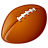# QlikView App Dev

Discussion Board for collaboration related to QlikView App Development.

Announcements
Customer & Partners, DEC. 9, 11 AM ET: Qlik Product & Strategy Roadmap Session: Data Analytics REGISTER NOW
cancel
Showing results for
Did you mean:Creator

## Expression Total Help

Hi All,

It's been awhile I haven't work on Qlikview. I come across expression total doesn't foot when dimension limits "show total"  are checked for Industry and Issuer.  What Im trying to do is to take "Sumproduct" column divide "Excluded Issuer" = Final Column. There are 3 Issuer been excluded, "J Soft", "Risk", and "Tan". Any information greatly appreciated!

Expected Value: for Issuer  and Industry Total:

Columns:

Sumproduct - expect to show 27,239 not 26,946 (not sure how this number comes in)

Excluded Issuer - Agree with 213,284

Final - expect to show 12.77111 % not 12.63366 %

Many Thanks,

Frank

1 Solution

Accepted SolutionsMVP

Sumproduct

=Sum(Aggr((sum({<[Flow Type]={'Cost','FX Hedging Loss'},Issuer-={'Risk','Tan','J Soft'}>}[Amount]) *-1/1000)

*

XIRR([Amount], [iDate]), Status, Industry, Issuer))

Final

=Sum(Aggr((sum({<[Flow Type]={'Cost','FX Hedging Loss'},Issuer-={'Risk','Tan','J Soft'}, [Status]=>}[Amount])*-1/1000)

*

XIRR([Amount], [iDate]), Status, Issuer, Industry))

/

(sum({<[Flow Type]={'Cost','FX Hedging Loss'},Issuer-={'Risk','Tan','J Soft'}>}[Amount])*-1/1000)

2 RepliesMVP

Sumproduct

=Sum(Aggr((sum({<[Flow Type]={'Cost','FX Hedging Loss'},Issuer-={'Risk','Tan','J Soft'}>}[Amount]) *-1/1000)

*

XIRR([Amount], [iDate]), Status, Industry, Issuer))

Final

=Sum(Aggr((sum({<[Flow Type]={'Cost','FX Hedging Loss'},Issuer-={'Risk','Tan','J Soft'}, [Status]=>}[Amount])*-1/1000)

*

XIRR([Amount], [iDate]), Status, Issuer, Industry))

/

(sum({<[Flow Type]={'Cost','FX Hedging Loss'},Issuer-={'Risk','Tan','J Soft'}>}[Amount])*-1/1000)Creator
Author

Thanks again Sunny!Community Browser